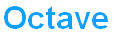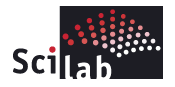# List of MATLAB open source alternatives

Need to work on some really complex math computations such as?

• Signal and image processing
• Financial modeling and analysis
• Algorithm development
• Data visualization
• Data analysis
• Numeric computation

Then MATLAB may be the perfect solution for you. To find out more about how MATLAB can benefit you, do check out this Free interactive MATLAB CD and Technical Kit Offer which includes product demos and webinars, technical kit containing data sheets for MATLAB and Signal Processing Toolbox, technical computing brochures and Mathworks Product Overview.

If MATLAB does not suit your needs or requirements, you may also consider the following list of MATLAB Open Source Alternatives with quite similar capabilities:GNU Octave is a high-level language, primarily intended for numerical computations. It provides a convenient command line interface for solving linear and nonlinear problems numerically, and for performing other numerical experiments using a language that is mostly compatible with Matlab. It may also be used as a batch-oriented language.

Octave has extensive tools for solving common numerical linear algebra problems, finding the roots of nonlinear equations, integrating ordinary functions, manipulating polynomials, and integrating ordinary differential and differential-algebraic equations. It is easily extensible and customizable via user-defined functions written in Octave’s own language, or using dynamically loaded modules written in C++, C, Fortran, or other languages.Scilab is an open source scientific software package for numerical computations providing a powerful open computing environment for engineering and scientific applications.

Scilab includes hundreds of mathematical functions with the possibility to add interactively programs from various languages (C, C++, Fortran). It has sophisticated data structures (including lists, polynomials, rational functions, linear systems), an interpreter and a high level programming language.

Some toolboxes available within the system includes 2D and 3D graphics / animation, linear algebra, sparse matrices, polynomials and rational functions, interpolation, approximation, simulation and many others.FreeMat is a free environment for rapid engineering and scientific prototyping and data processing. It is similar to commercial systems such as MATLAB from Mathworks, and IDL from Research Systems, but is Open Source. FreeMat is available under the GPL license.

In addition to supporting many MATLAB functions and some IDL functionality, it features codeless interface to external C, C++, and Fortran code, further parallel distributed algorithm development (via MPI), and some extended volume and 3D visualization capabilities.Sage is a free open-source mathematics software system licensed under the GPL. It combines the power of many existing open-source packages into a common Python-based interface. Sage’s mission is to create a viable free open source alternative to Magma, Maple, Mathematica and Matlab.

Sage is free open source math software that supports research and teaching in algebra, geometry, number theory, cryptography, and related areas. Both the Sage development model and the technology in Sage itself are distinguished by an extremely strong emphasis on openness, community, cooperation, and collaboration. Sage claims to be building the car rather than reinventing the wheel.

Sage can be used in several ways – notebook graphical interface, interactive command line, programs and scripts.SciPy is an Open Source library of scientific tools for Python. It depends on the NumPy library, and it gathers a variety of high level science and engineering modules together as a single package. SciPy provides modules for statistics,optimization, numerical integration,linear algebra, Fourier transforms, signal processing,image processing, genetic algorithms, ODE solvers, special functions, and more.

SciPy is developed concurrently on both Linux and Windows. It should also compile and run successfully on Mac, Solaris, FreeBSD, and most other platforms where Python is available.

———————— End ——————————-

There you go, 5 MATLAB Open Source Alternatives for you to evaluate and start to complex calculations.